# The SEQDESIGN Procedure

### Applicable Regression Parameter Tests and Sample Size Computation

Subsections:

The SEQDESIGN procedure provides sample size computation for tests of a regression parameter in three regression models: normal regression, logistic regression, and proportional hazards regression.

To test a parameter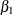in a regression model, the variance of the parameter estimateis needed for the sample size computation. In a simple regression model with one covariate X1, the variance ofis inversely related to the variance of X1,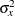. That is,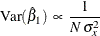for the normal regression and logistic regression models, where N is the sample size, andfor the proportional hazards regression model, where D is the number of events.

For a regression model with more than one covariate, the variance offor the normal regression and logistic regression models is inversely related to the variance of X1 after adjusting for other covariates. That is,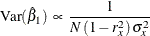whereis the estimate of the parameterin the model and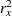is the R square from the regression of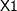on other covariates—that is, the proportion of the varianceexplained by these covariates.

Similarly, for a proportional hazards regression model,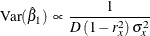Thus, with the derived maximum information, the required sample size or number of events can also be computed for the testing of a parameter in a regression model with covariates.

#### Test for a Parameter in the Regression Model

The MODEL=REG option in the SAMPLESIZE statement derives the sample size required for a Z test of a normal regression. For a normal linear regression model, the response variable is normally distributed with the mean equal to a linear function of the explanatory variables and the constant variance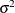.

The normal linear model is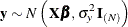where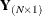is the vector of the N observed responses,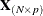is the design matrix for these N observations,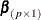is the parameter vector, and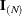is the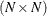identity matrix.

The least squares estimate is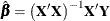and is normally distributed with mean
and variance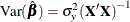For a model with only one covariate X1,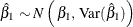where the variance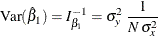Thus, with the derived maximum information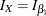, the required sample size is given by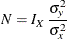For a normal linear model with more than one covariate, the variance of a single parameteris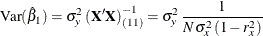where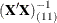is the diagonal element of the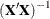matrix corresponding to the parameter,is the variance of the variable X1, andis the proportion of variance of X1 explained by other covariates. The value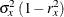represents the variance of X1 after adjusting for all other covariates.

Thus, with the derived maximum information
, the required sample size is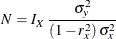In the SEQDESIGN procedure, you can specify the MODEL=REG( VARIANCE=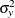XVARIANCE=XRSQUARE=) option in the SAMPLESIZE statement to compute the required total sample size and individual sample size at each stage. A SAS procedure such as PROC REG can be used to compute the parameter estimate and its standard error at each stage.

#### Test for a Parameter in the Logistic Regression Model

The MODEL=LOGISTIC option in the SAMPLESIZE statement derives the sample size required for a Z test of a logistic regression parameter. The linear logistic model has the form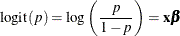where p is the response probability to be modeled andis a vector of parameters.

Following the derivation in the section Test for a Parameter in the Regression Model, the required sample size for testing a parameter inis given byWith the variance of the logit response,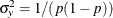,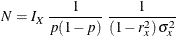whereis the variance of X andis the proportion of variance explained by other covariates.

In the SEQDESIGN procedure, you can specify the MODEL=LOGISTIC( PROP=p XVARIANCE=XRSQUARE=) option in the SAMPLESIZE statement to compute the required total sample size and individual sample size at each stage.

A SAS procedure such as PROC LOGISTIC can be used to compute the parameter estimate and its standard error at each stage.

#### Test for a Parameter in the Proportional Hazards Regression Model

The MODEL=PHREG option in the SAMPLESIZE statement derives the number of events required for a Z test of a proportional hazards regression parameter. For analyses of survival data, Cox’s semiparametric model is often used to examine the effect of explanatory variables on hazard rates. The survival time of each observation in the population is assumed to follow its own hazard function,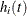, expressed as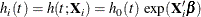where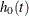is an arbitrary and unspecified baseline hazard function,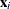is the vector of explanatory variables for the ith individual, andis the vector of regression parameters associated with the explanatory variables.

Hsieh and Lavori (2000, p. 553) show that the required number of events for testing a parameter in,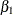, associated with the variable X1 is given by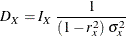whereis the variance of X1 andis the proportion of variance of X1 explained by other covariates.

In the SEQDESIGN procedure, you can specify the MODEL=PHREG( XVARIANCE=XRSQUARE=) option in the SAMPLESIZE statement to compute the required number of events and individual number of events at each stage.

A SAS procedure such as PROC PHREG can be used to compute the parameter estimate and its standard error at each stage.

Note that for a two-sample test, X1 is an indicator variable and is the only covariate in the model. Thus, if the two sample sizes are equal, then the variance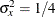and the required number of events for testing the parameteris given by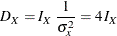See the section Input Number of Events for Fixed-Sample Design for a detailed description of the sample size computation that uses hazard rates, accrual rate, and accrual time.